Question

# If a gas occupies 2.0 L at 0.0 °C, what is the volume at 273 °C...

If a gas occupies 2.0 L at 0.0 °C, what is the volume at 273 °C if the pressure does not change?

By Charles' law, at constant pressure

Volume of a gas (V) is directly proportional to absolute temperature (T).

Or,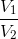=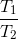Given, V1 = 2.0 L

T1 = (0.0+273.15 ) K = 273.15 K.

T2 = (273+273.15) K = 546.15 K.

Then, V2 =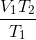Or, V2 = (2.0 × 546.15/273.15) L

= 4.0 L ( 2 significant figures).

So, volume at 2730c is 4.0 L.

#### Earn Coins

Coins can be redeemed for fabulous gifts.

Similar Homework Help Questions
• ### A piston at 1.1 atm contains a gas that occupies a volume of 3.5 L. What...

A piston at 1.1 atm contains a gas that occupies a volume of 3.5 L. What pressure would have to be placed on the piston to force the volume to adjust to 0.38 L? (b) A piston at 40.0°C contains a gas that occupies a volume of 2.5 L. To what temperature would the gas have to be heated to increase the volume to 7.4 L at constant pressure? (c) A piston at 445 torr contains a gas that occupies...

• ### T = 273 T2=352 C + 273./ SEUDUB What will be the volume at STP of...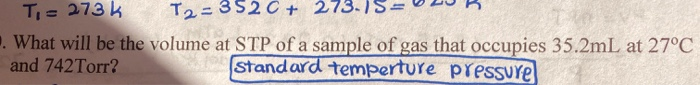T = 273 T2=352 C + 273./ SEUDUB What will be the volume at STP of a sample of gas that occupies 35.2mL at 27°C and 742 Torr? Standard temperture pressure

• ### A sample of gas has a volume of 3.0 L at a pressure of 2.0 atm....

A sample of gas has a volume of 3.0 L at a pressure of 2.0 atm. What is the volume of the gas in L at 10.0 atm? Temperature is constant. A sample of gas has a volume of 20.0 L at a pressure of 1.0 atm. What is the volume of the gas in L at 5.0 atm? Temperature is constant. A sample of gas has a volume of 4.0 L at a pressure of 0.50 atm. What is...

• ### 1.  If a gas occupies 2 L at 3 atm, how many liters will it occupy at...

1.  If a gas occupies 2 L at 3 atm, how many liters will it occupy at 6 atm if the temperature is not changed? a)1L b)2L c)3L d)4L 2. IF A GAS OCCUPIES 2 L AT 273 °C, WHAT IS THE VOLUME AT 0 °C IF THE PRESSURE DOES NOT CHANGE? a)0.5 L b)1 L c)2 L d)4 L 3. If a gas occupies 5 L at 298 K, what temperature is needed to reduce the volume to 2 L...

• ### (a) A piston at 6.8 at contains a gas that occupies a volume of 3.5 L...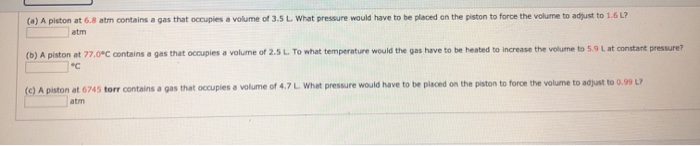(a) A piston at 6.8 at contains a gas that occupies a volume of 3.5 L What pressure would have to be placed on the piston to force the volume to adjust to 1.6L? (D) A piston at 77.0°C contains a gas that occupies a volume of 2.5 L To what temperature would the gas have to be heated to increase the volume to 5.9 Lat constant pressure? (c) A piston at 6745 torr contains a gas that occupies a...

• ### 1.0 g sample of a diatomic gas occupies a volume of 350 mL at 0.0*C and...

1.0 g sample of a diatomic gas occupies a volume of 350 mL at 0.0*C and 2.0 atm. Find the mass of one atom of the gas. A. 2.7 X 10^23g B. 2.7 X 10^20g C. 1.4 X 10^23g D. 1.4 X 10^20g

• ### A sample of a gas at room temperature occupies a volume of 35.0 L at a...

A sample of a gas at room temperature occupies a volume of 35.0 L at a pressure of 792 torr . If the pressure changes to 3960 torr , with no change in the temperature or moles of gas, what is the new volume, V2? If the volume of the original sample in Part A (P1 = 792 torr , V1 = 35.0 L ) changes to 67.0 L , without a change in the temperature or moles of gas...

• ### An ideal gas with γ= 1.5 occupies a volume of V0= 2.0 L. The gas expands...

An ideal gas with γ= 1.5 occupies a volume of V0= 2.0 L. The gas expands isothermally to a volumeof V= 6.8 L. Alternatively, the gas reaches the same final state by firstadiabatically expanding to thefinal volume then heating isochorically to the final temperature. For which path is the heat transferredto the gas greatest and by what percent of the lesser amount is it greater?

• ### (a) A piston at 4.9 atm contains a gas that occupies a volume of 3.5 L....

(a) A piston at 4.9 atm contains a gas that occupies a volume of 3.5 L. What pressure would have to be placed on the piston to force the volume to adjust to 0.81 L? atm (b) A piston at 81.0°C contains a gas that occupies a volume of 2.5 L. To what temperature would the gas have to be heated to increase the volume to 7.1 L at constant pressure? °C (c) A piston at 7345 torr contains a...

• ### 5. If a sample of gas occupies 6.80 L at 325°C, what will its volume be...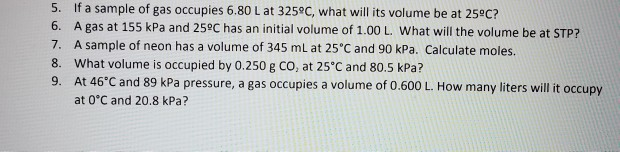5. If a sample of gas occupies 6.80 L at 325°C, what will its volume be at 25°C? 6. A gas at 155 kPa and 25°C has an initial volume of 1.00 L. What will the volume be at STP? 7. A sample of neon has a volume of 345 mL at 25°C and 90 kPa. Calculate moles. 8. What volume is occupied by 0.250 g CO, at 25°C and 80.5 kPa? 9. At 46°C and 89 kPa pressure, a...